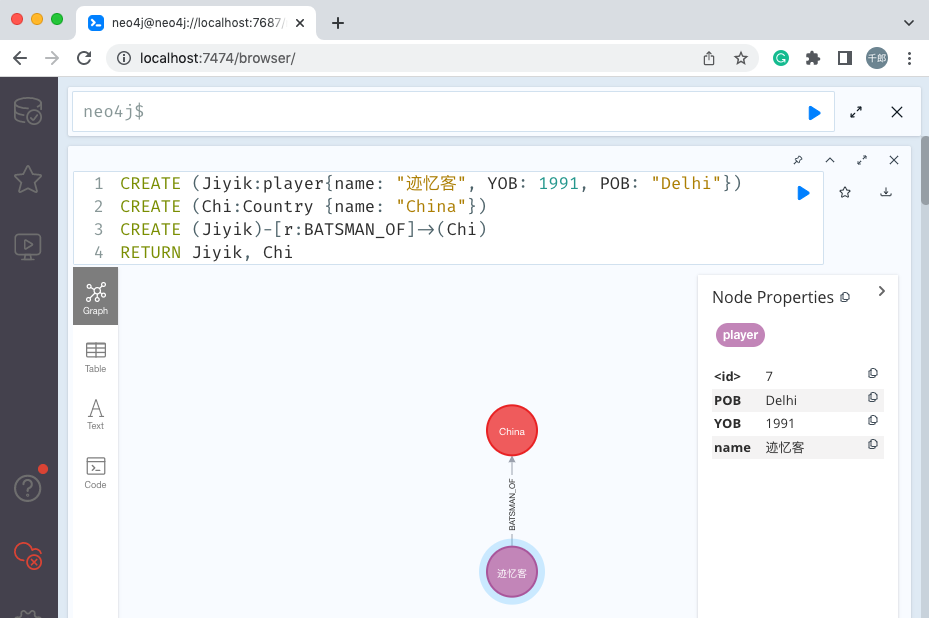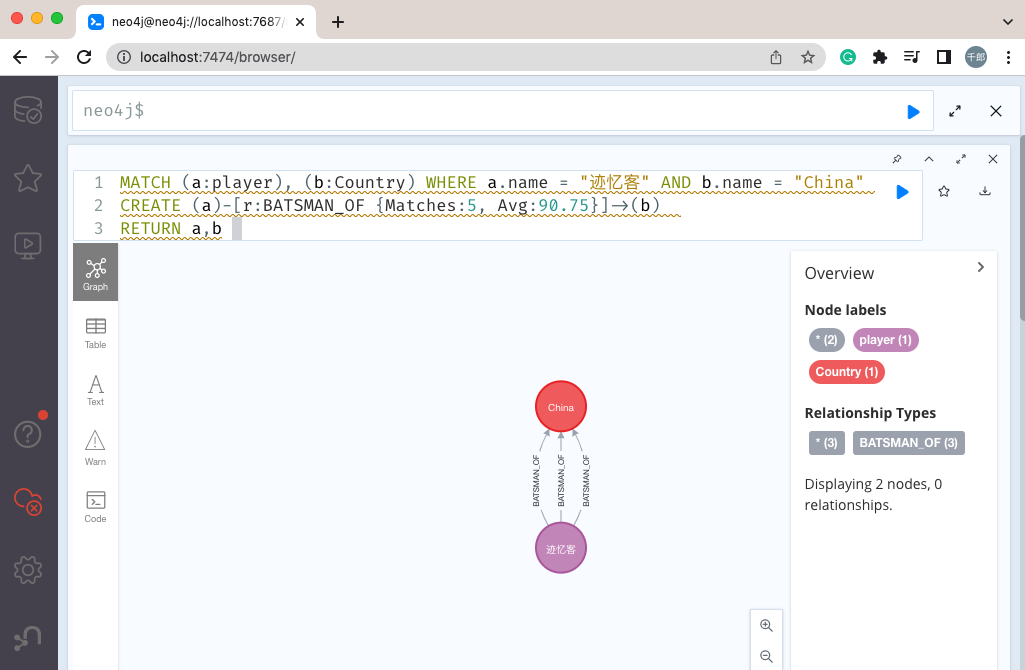# Neo4j 创建关系

• 建立关系
• 在现有节点之间创建关系
• 使用标签和属性创建关系

## 建立关系

### 语法

``````CREATE (node1)-[:RelationshipType]->(node2)
``````

### 示例

``````CREATE (Jiyik:player{name: "迹忆客", YOB: 1991, POB: "Delhi"})
CREATE (Chi:Country {name: "China"})
``````

``````CREATE (Jiyik)-[r:BATSMAN_OF]->(Chi)
``````

``````RETURN Jiyik, Chi
``````## 在现有节点之间创建关系

### 语法

``````MATCH (a:LabeofNode1), (b:LabeofNode2)
WHERE a.name = "nameofnode1" AND b.name = " nameofnode2"
CREATE (a)-[: Relation]->(b)
RETURN a,b
``````

### 示例

``````MATCH (a:player), (b:Country) WHERE a.name = "迹忆客" AND b.name = "China"
CREATE (a)-[r: BATSMAN_OF]->(b)
RETURN a,b
``````## 使用标签和属性创建关系

### 语法

``````CREATE (node1)-[label:Rel_Type {key1:value1, key2:value2, . . . n}]-> (node2)
``````

### 示例

``````MATCH (a:player), (b:Country) WHERE a.name = "迹忆客" AND b.name = "China"
CREATE (a)-[r:BATSMAN_OF {Matches:5, Avg:90.75}]->(b)
RETURN a,b
``````## 创建完整路径

### 语法

``````CREATE p = (Node1 {properties})-[:Relationship_Type]->
(Node2 {properties})[:Relationship_Type]->(Node3 {properties})
RETURN p
``````

### 示例

``````CREATE p = (Jiyik {name:"迹忆客"}) - [:TOPSCORRER_OF]->
(Chi{name:"China"}) - [:WINNER_OF] -> (CT2013{name:"Champions Trophy2013"})
RETURN p
``````##  查看笔记